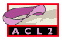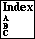O-RST

returns the rest of an infinite ordinal
```Major Section:  PROGRAMMING
```

An ACL2 infinite ordinal is a list whose elements are exponent-coefficient pairs (see o-p and see o-infp). The first exponent and first coefficient of an ordinal can be obtained by using `o-first-expt` and `o-first-coeff` respectively. To obtain the rest of the ordinal (for recursive analysis), use the `o-rst` function. It returns the rest of the ordinal after the first exponent and coefficient are removed.